# Forward-Backward Splitting¶

$\newcommand{\dotp}{\langle #1, #2 \rangle}$ $\newcommand{\enscond}{\lbrace #1, #2 \rbrace}$ $\newcommand{\pd}{ \frac{ \partial #1}{\partial #2} }$ $\newcommand{\umin}{\underset{#1}{\min}\;}$ $\newcommand{\umax}{\underset{#1}{\max}\;}$ $\newcommand{\umin}{\underset{#1}{\min}\;}$ $\newcommand{\uargmin}{\underset{#1}{\arg\min}\;}$ $\newcommand{\norm}{\|#1\|}$ $\newcommand{\abs}{\left|#1\right|}$ $\newcommand{\choice}{ \left\{ \begin{array}{l} #1 \end{array} \right. }$ $\newcommand{\pa}{\left(#1\right)}$ $\newcommand{\diag}{{diag}\left( #1 \right)}$ $\newcommand{\qandq}{\quad\text{and}\quad}$ $\newcommand{\qwhereq}{\quad\text{where}\quad}$ $\newcommand{\qifq}{ \quad \text{if} \quad }$ $\newcommand{\qarrq}{ \quad \Longrightarrow \quad }$ $\newcommand{\ZZ}{\mathbb{Z}}$ $\newcommand{\CC}{\mathbb{C}}$ $\newcommand{\RR}{\mathbb{R}}$ $\newcommand{\EE}{\mathbb{E}}$ $\newcommand{\Zz}{\mathcal{Z}}$ $\newcommand{\Ww}{\mathcal{W}}$ $\newcommand{\Vv}{\mathcal{V}}$ $\newcommand{\Nn}{\mathcal{N}}$ $\newcommand{\NN}{\mathcal{N}}$ $\newcommand{\Hh}{\mathcal{H}}$ $\newcommand{\Bb}{\mathcal{B}}$ $\newcommand{\Ee}{\mathcal{E}}$ $\newcommand{\Cc}{\mathcal{C}}$ $\newcommand{\Gg}{\mathcal{G}}$ $\newcommand{\Ss}{\mathcal{S}}$ $\newcommand{\Pp}{\mathcal{P}}$ $\newcommand{\Ff}{\mathcal{F}}$ $\newcommand{\Xx}{\mathcal{X}}$ $\newcommand{\Mm}{\mathcal{M}}$ $\newcommand{\Ii}{\mathcal{I}}$ $\newcommand{\Dd}{\mathcal{D}}$ $\newcommand{\Ll}{\mathcal{L}}$ $\newcommand{\Tt}{\mathcal{T}}$ $\newcommand{\si}{\sigma}$ $\newcommand{\al}{\alpha}$ $\newcommand{\la}{\lambda}$ $\newcommand{\ga}{\gamma}$ $\newcommand{\Ga}{\Gamma}$ $\newcommand{\La}{\Lambda}$ $\newcommand{\si}{\sigma}$ $\newcommand{\Si}{\Sigma}$ $\newcommand{\be}{\beta}$ $\newcommand{\de}{\delta}$ $\newcommand{\De}{\Delta}$ $\newcommand{\phi}{\varphi}$ $\newcommand{\th}{\theta}$ $\newcommand{\om}{\omega}$ $\newcommand{\Om}{\Omega}$

In :
library(pracma)
# library(SynchWave)

# Importing the libraries
for (f in list.files(path="nt_toolbox/toolbox_general/", pattern="*.R")) {
source(paste("nt_toolbox/toolbox_general/", f, sep=""))
}
for (f in list.files(path="nt_toolbox/toolbox_signal/", pattern="*.R")) {
source(paste("nt_toolbox/toolbox_signal/", f, sep=""))
}
for (f in list.files(path="nt_toolbox/toolbox_graph/", pattern="*.R")) {
source(paste("nt_toolbox/toolbox_graph/", f, sep=""))
}


This numerical tour presents the Forward-Backward (FB) algorithm to minimize the sum of a smooth and a simple function. It shows an application to sparse deconvolution.

## Forward-Backward Algorithm¶

We consider the problem of minimizing the sum of two functions $$E^\star = \umin{x \in \RR^N} E(x) = f(x) + g(x).$$

So, we want to find a vector $x^{\star}$ solution to the problem, i.e. a minimizer of $E=f+g$.

We assume that $f$ is a $C^1$ function with $L$-Lipschitz gradient.

We also assume that $g$ is "simple", in the sense that one can compute exactly and quickly its proximity operator, which is defined as $$\text{prox}_{\ga g}(x) = \uargmin{y \in \RR^N} \frac{1}{2}\norm{x-y}^2 + \ga g(y).$$ for any $\ga > 0$.

The forward-backward algorithm reads, after initializing $x^{(0)} \in \RR^N$, $$x^{(k+1)} = \text{prox}_{\ga g}\pa{ x^{(k)} - \ga \nabla f( x^{(k)} ) }.$$

If $0 < \ga < \frac{2}{L}$, then this scheme converges to a minimizer of $f+g$.

## Sparse Regularization of Inverse Problems¶

We consider a linear inverse problem $$y = \Phi x_{0} + w \in \RR^P$$ where $x_{0} \in \RR^N$ is the (unknown) signal to recover, $w \in \RR^P$ is a noise vector, and $\Phi \in \RR^{P \times N}$ models the acquisition device.

To recover an estimate of the signal $x_{0}$, we consider basis pursuit denoising, which makes use of the $\ell^1$ norm as sparsity enforcing penalty: $$\umin{x \in \RR^N} \frac{1}{2} \norm{\Phi x-y}^2 + \la \norm{x}_1,$$ where the $\ell^1$ norm is defined as $$\norm{x}_1 = \sum_i \abs{x_i}.$$

The parameter $\la$ should be set in accordance to the noise level $\norm{w}$.

This minimization problem can be cast in the form of minimizing $f+g$ where $$f(x) = \frac{1}{2} \norm{\Phi x-y}^2 \qandq g(x) = \la \norm{x}_1.$$

$f$ is smooth; we have $$\nabla f(x) = \Phi^{*} (\Phi x - y),$$ which is $L$-Lipschitz continuous, with $$L = \norm{ \Phi^* \Phi }.$$

The $\ell^1$-norm is "simple", because its proximal operator is soft thresholding: $$\big(\text{prox}_{\ga g}(x)\big)_n = \max\pa{ 0, 1 - \frac{\la \ga}{\abs{x_n}} } x_n.$$

## Signal Deconvolution on Synthetic Sparse Data¶

A simple linearized model of seismic acquisition considers a linear filtering operator (convolution): $$\Phi x = \phi \ast x$$

The filter $\phi$ is called the impulse response, or the poind spread function, of the acquisition process $x\mapsto \Phi x$.

In :
N = 1024


We define the width of the filter.

In :
s = 5


Second derivative of a Gaussian.

In :
t = c(-(N/2) : ((N/2) - 1))
h = (1-t**2/s**2)*exp(-(t**2)/(2*s**2))
h = h - mean(h)

In :
fftshift = SynchWave::fftshift
h1 = fftshift(h) # Recenter the filter for fft use.
Phi = function (u) {Re(ifft(fft(h1) * fft(c(u))))}


We display the filte and its spectrum (amplitude of its Fourier transform).

In :
# Fourier transform (normalized)
hf = Re(pracma::fftshift(fft(h1))) / sqrt(N)

options(repr.plot.width=5, repr.plot.height=3.5)

q = 200

plot(t, h, type="l", main="Filter, Spacial (zoom)",
col="blue", xlim=c(-200,200), ylab="")

plot(t, hf, type="l",
main="Filter, Fourrier (zoom)", col="blue",
xlim=c(-200,200), ylab="")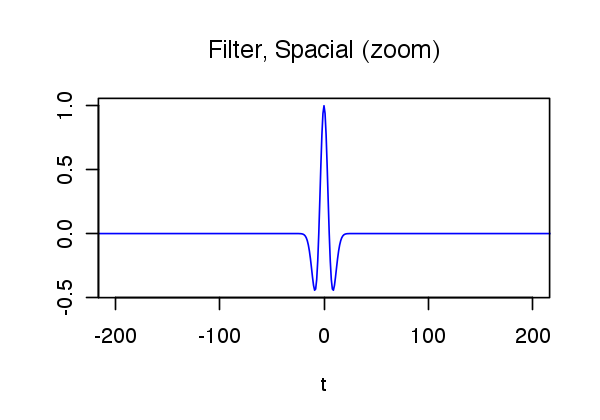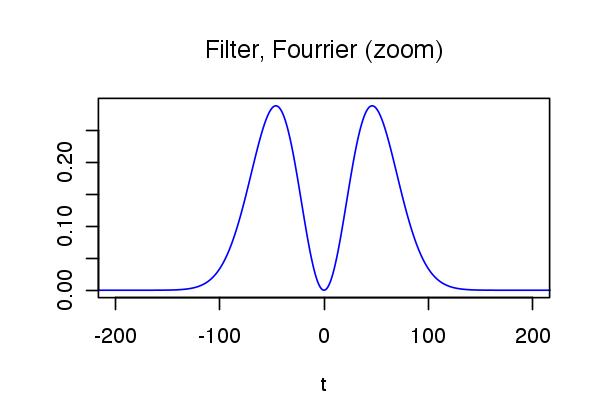We generate a synthetic sparse signal $x_{0}$, with only a small number of nonzero coefficients.

Number of Diracs of the signal.

In :
s = round(N * 0.03)


Set the seed-number (for reproductibility).

In :
set.seed(1)


Location of the diracs.

In :
sel = sample(c(1 : N))
sel = sel[0:s]


Signal $x_{0}$.

In :
x0 = zeros(1, N)
x0[sel] = 1.
x0 = x0 * sign(randn(1, N)) * (1 - .3 * rand(1, N))


Noise level.

In :
sigma = 0.06


Compute the measurements $y= \Phi x_{0} + w$ where $w$ is a realization of white Gaussian noise of variance $\sigma^{2}$.

In :
y = Phi(x0) + sigma * randn(1, N)

In :
stemplot(x0, col="blue", ylab="", xlab="", main="Signal")
plot(c(1:N), y, xlab="", ylab="", col="blue", main="Measurements y", type="l")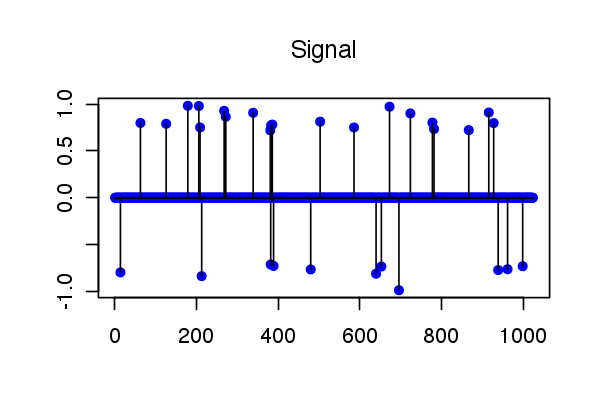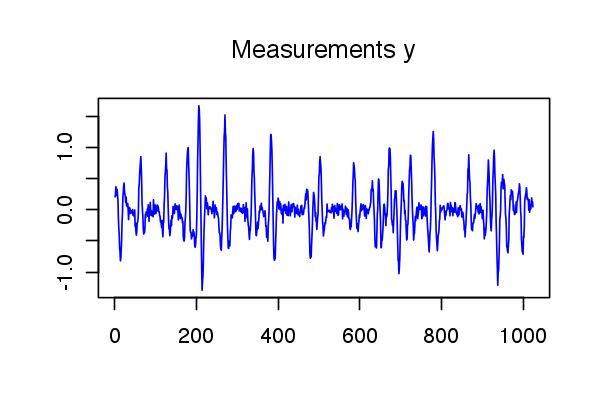## Deconvolution¶

We now implement the foward-backward algorithm to recover an estimate of the sparse signal

We define the regularization parameter $\la$.

In :
Lambda = 1.


We define the proximity operator of $\ga g$.

In :
proxg = function(x, gamma, Lambda) {x * pmax(1 - ((Lambda * gamma) / abs(x)), 0)}


We define the gradient operator of $f$. Note that $\Phi^*=\Phi$ because the filter $\phi$ is symmetric.

Exercise 1

Write the code of the function grad_f.

In :
source("nt_solutions/optim_2_condat_fb/exo1.R")

In :
# Insert your code here.


We define the Lipschitz constant $\beta$ of $\nabla f$.

In :
L = max(abs(fft(h)))**2


We define the stepsize $\ga$, which must be smaller than $2/\beta$.

In :
gamma = 1.95 / L


We compute the solution of $\ell_1$ deconvolution (basis pursuit denoising). We keep track of the energy $E_k=f(x^{(k)})+g(x^{(k)})$.

Exercise 2

Write the code of the forward-backward iteration.

In :
source("nt_solutions/optim_2_condat_fb/exo2.R")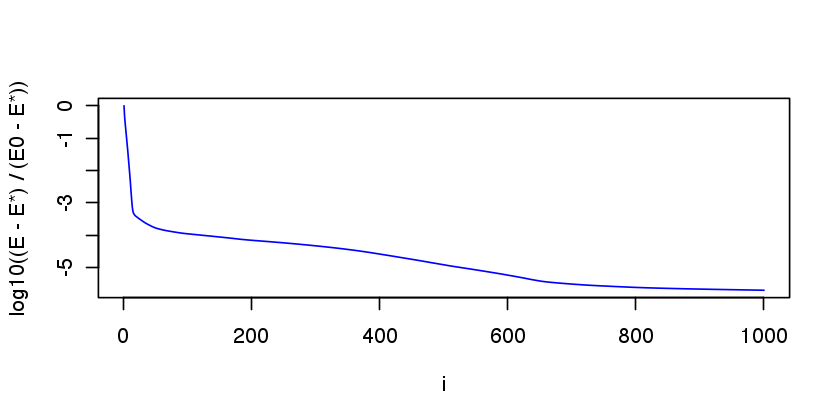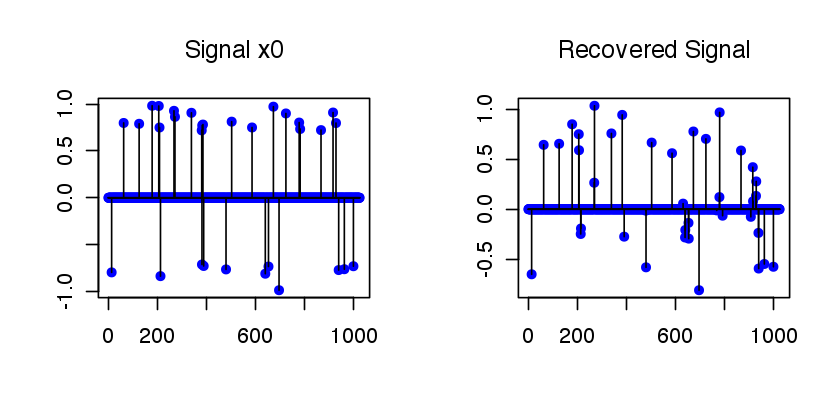In :
# Insert code here.


We display the result.

In :
# Insert code here.


We plot the relative error $(E_k-E^\star)/(E_0-E^\star)$ in log-scale with respect to $k$.

In :
# Insert code here.


## Over-relaxed Forward-Backward¶

It is possible to introduce a relaxation parameter $\rho$ with $0 < \rho < 1$. The over-relaxed foward-backward algorithm initializes $x^{(0)} \in \RR^N$, and then iterates, for $k=1,2,\ldots$ $$z^{(k)} = \text{prox}_{\ga g}\pa{ x^{(k-1)} - \ga \nabla f( x^{(k-1)} ) }.$$ $$x^{(k)} = z^{(k)} + \rho \pa{ z^{(k)} - x^{(k-1)} }$$

Let us assume $\gamma=1/\beta$. Convergence of the iterates $x^{(k)}$ and $z^{(k)}$ to a solution is guaranteed for $0 < \rho < 1/2$. The weaker property of convergence of $E(x^{(k)})$ to $E^\star$ is proved, when $1/2\leq \rho <1$.

In :
gamma = 1 / L


Exercise 3

Write the code of the over-relaxed forward-backward iteration.

In :
source("nt_solutions/optim_2_condat_fb/exo3.R")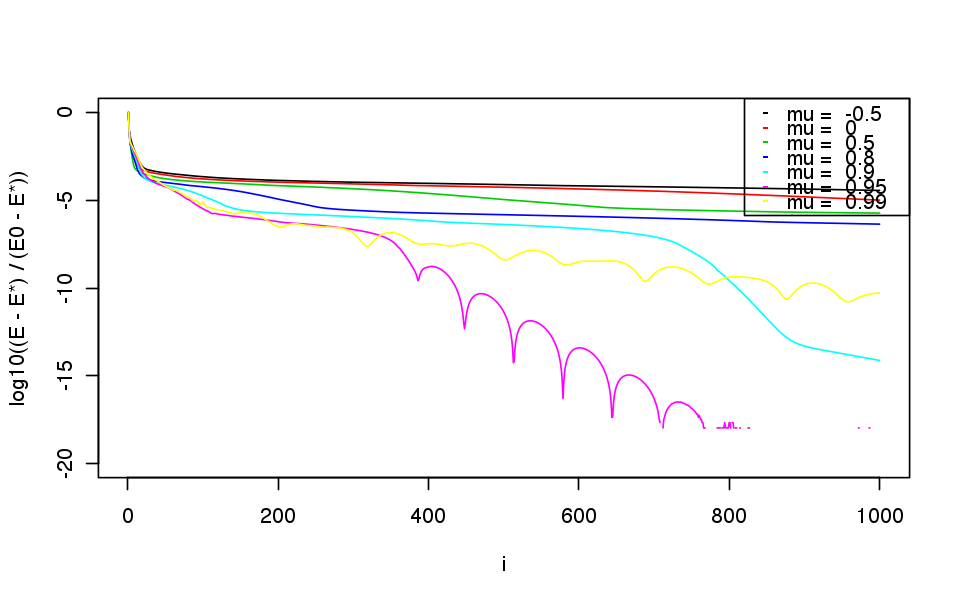In :
# Insert code here.


As we see, in this example, over-relaxation does not bring any speedup, because $\gamma$ is lower than without over-relaxation. There are other setting parameters or other problems, for which over-relaxation does bring a significant speedup.

## FISTA-like Accelerated Forward-Backward Algorithm¶

We consider the FISTA algorithm introduced in:

A. Beck and M. Teboulle, "A Fast Iterative Shrinkage-Thresholding Algorithm for Linear Inverse Problems", SIAM Journal on Imaging Sciences, 2009.

More precisely, we consider a slightly modified version of FISTA, whose convergence is proved, see A. Chambolle and C. Dossal, "On the convergence of the iterates of "FISTA"", preprint, 2015.

Given an initial estimate $x^{(0)}$ of the solution and a parameter $a>2$, the algorithm sets $\gamma=1/\beta$, sets $z^{(0)}=x^{(0)} \in \RR^N$, and iterates, for $k=1,2,\ldots$ $$x^{(k)} = \text{prox}_{\ga g}\pa{ z^{(k-1)} - \ga \nabla f( z^{(k-1)} ) }.$$ $$\alpha_k=(k-1)/(k+a)$$ $$z^{(k)} = x^{(k)} + \alpha_k \pa{ x^{(k)} - x^{(k-1)} }$$

It is proved that the iterates $x^{(k)}$ converge to a solution $x^\star$ of the problem. Moreover, the optimal convergence rate for this class of problems is reached, namely $$E_k - E^\star = O(1/k^2),$$ whereas the convergence rate for the normal forward-backward is only $O(1/k)$.

Note the difference between the over-relaxed forward-backward and the accelerated forward-backward: the later is based on an inertia mechanism, of different nature than over-relaxation.

Exercise 4

Write the code of the accelerated forward-backward iteration.

In :
source("nt_solutions/optim_2_condat_fb/exo4.R")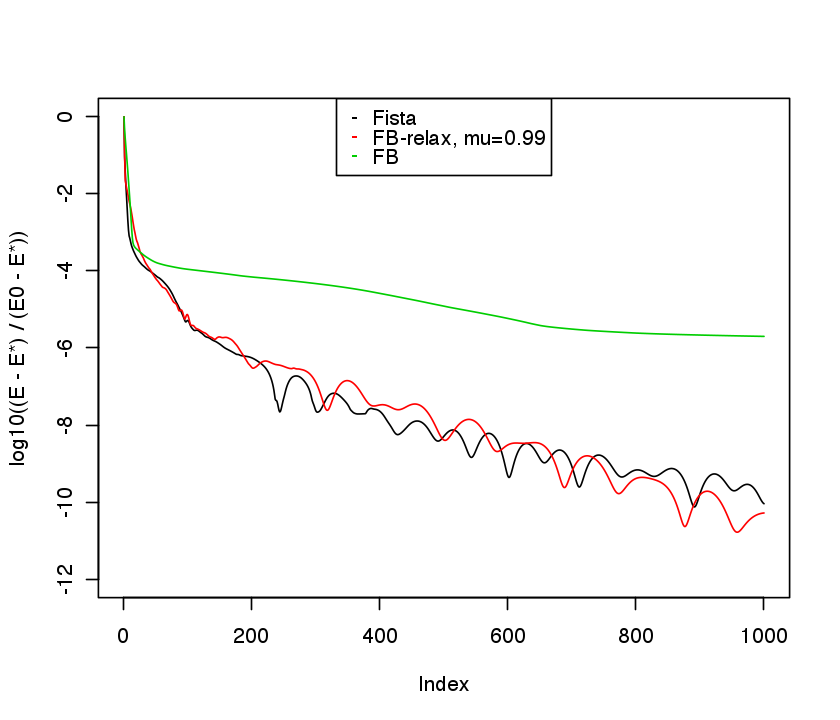In :
# Insert code here.


We can note that the accelerated forward-backward is not monotonic: the cost function E is not decreasing along with the iterations and some oscillations are present.